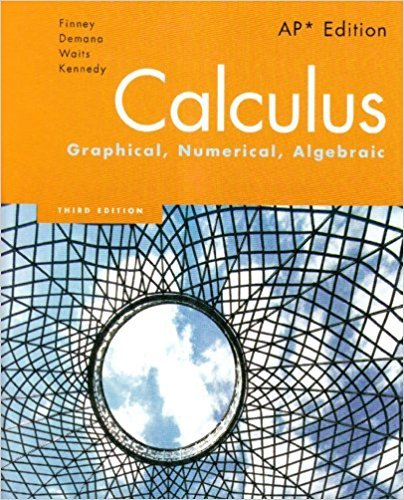×
×

# Solutions for Chapter 7: Calculus: Graphical, Numerical, Algebraic 3rd Edition## Full solutions for Calculus: Graphical, Numerical, Algebraic | 3rd Edition

ISBN: 9780132014083Solutions for Chapter 7

Solutions for Chapter 7
4 5 0 356 Reviews
13
3
##### ISBN: 9780132014083

This expansive textbook survival guide covers the following chapters and their solutions. Calculus: Graphical, Numerical, Algebraic was written by and is associated to the ISBN: 9780132014083. Chapter 7 includes 55 full step-by-step solutions. This textbook survival guide was created for the textbook: Calculus: Graphical, Numerical, Algebraic, edition: 3. Since 55 problems in chapter 7 have been answered, more than 4527 students have viewed full step-by-step solutions from this chapter.

Key Calculus Terms and definitions covered in this textbook
• Angle of elevation

The acute angle formed by the line of sight (upward) and the horizontal

• Component form of a vector

If a vector’s representative in standard position has a terminal point (a,b) (or (a, b, c)) , then (a,b) (or (a, b, c)) is the component form of the vector, and a and b are the horizontal and vertical components of the vector (or a, b, and c are the x-, y-, and z-components of the vector, respectively)

• Cotangent

The function y = cot x

• Directed line segment

See Arrow.

• Eccentricity

A nonnegative number that specifies how off-center the focus of a conic is

• End behavior asymptote of a rational function

A polynomial that the function approaches as.

• Endpoint of an interval

A real number that represents one “end” of an interval.

• equation of an ellipse

(x - h2) a2 + (y - k)2 b2 = 1 or (y - k)2 a2 + (x - h)2 b2 = 1

• Focal axis

The line through the focus and perpendicular to the directrix of a conic.

• Frequency distribution

See Frequency table.

• Graph of parametric equations

The set of all points in the coordinate plane corresponding to the ordered pairs determined by the parametric equations.

• Independent events

Events A and B such that P(A and B) = P(A)P(B)

• Permutations of n objects taken r at a time

There are nPr = n!1n - r2! such permutations

• Principle of mathematical induction

A principle related to mathematical induction.

• Random numbers

Numbers that can be used by researchers to simulate randomness in scientific studies (they are usually obtained from lengthy tables of decimal digits that have been generated by verifiably random natural phenomena).

• Removable discontinuity at x = a

lim x:a- ƒ(x) = limx:a+ ƒ(x) but either the common limit is not equal ƒ(a) to ƒ(a) or is not defined

• Residual

The difference y1 - (ax 1 + b), where (x1, y1)is a point in a scatter plot and y = ax + b is a line that fits the set of data.

• Right triangle

A triangle with a 90° angle.

• Subtraction

a - b = a + (-b)

• Terminal point

See Arrow.

×